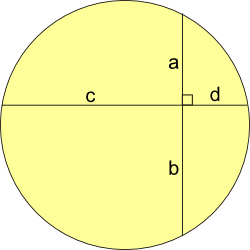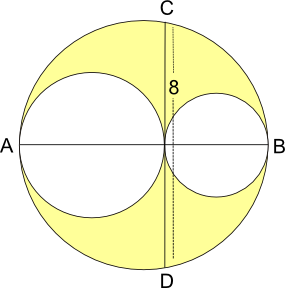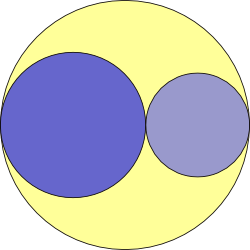You may also likeFitting In

The largest square which fits into a circle is ABCD and EFGH is a square with G and H on the line CD and E and F on the circumference of the circle. Show that AB = 5EF. Similarly the largest equilateral triangle which fits into a circle is LMN and PQR is an equilateral triangle with P and Q on the line LM and R on the circumference of the circle. Show that LM = 3PQLook Before You Leap

Can you spot a cunning way to work out the missing length?Triangle Midpoints

You are only given the three midpoints of the sides of a triangle. How can you construct the original triangle?

Partly Circles

Age 14 to 16Challenge Level

Here are three problems involving circles.

Can you solve them?
Can you find relationships between the three problems?

Firstly Show that ab = cd (where a, b, c, d are lengths)Secondly:

These three circles are drawn so that they touch each other, and their centres are all on the line AB.

If CD is $8$ units in length, what is the area of the region shaded yellow?Lastly: If the area shaded yellow is equal to the area of the larger of the two blue circles, what is the relationship between the radii of the three circles?